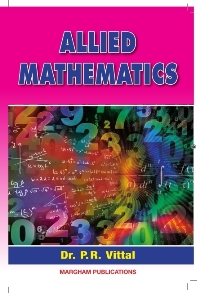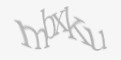24, Ground Floor, Rameswaram Road, T.Nagar, Chennai -600 017. Phone: 044 2432 246

#### Best Customer Support

Call:044 22501105 (Guindy),
044 24322469 (T. Nagar)

#### Reasonable price## Best Sellers

Shop nowShop now

# Allied Mathematics (for B.Sc. and B.C.A. for all Indian Universities in Single Volume) - P.R. Vittal• Partial Fractions
• Binomial Series
• Exponential Series
• Logarithmic Series
• Matrices
• Theory of Equations
• Finite Differences
• Successive Differentiation - nth Derivatives
• Partial Differentiation
• Jacobians
• Maxima and Minima of functions of two variables
• Lagrange's Multiples
• Polar Co-ordinates (Calculus)
• Curvature and Radius of curvature
• Polar Co-ordinates
• Curve Tracing
• Trigonometry
• Expansions
• Roots of Equations
• Hyperbolic functions
• Logrithms of complex numbers
• Gregory's Series
• Summation of Series
• Integration
• Reduction Formula
• Integration as Limit of Summation
• Length of an Arc
• Surface Area
• Multiple Integrals
• Fourier Series
• Differential Equations of First order and Higher degree
• Second order Differential Equations with constant coefficients
• Linear Homogeneous equation and variation of parameter
• Total Differential Equations
• Partial Differential Equations
• Laplace Transform
• Vector Differentiation
• Vector Integration
• Beta, Gamma Functions
Book Author P.R. Vittal Margham Publications English B.Sc. and BCA for all Indian Universities in single Volume.₹350.00
Email to friend